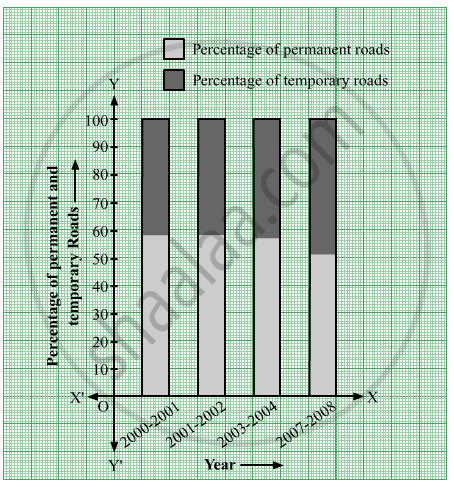Share

# In the Table Given Below, the Information is Given About Roads. Using this Draw Sub-divided and Percentage Bardiagram - Algebra

ConceptBar Diagrams

#### Question

In the table given below, the information is given about roads. Using this draw sub-divided and percentage bardiagram (Approximate the percentages to the nearest integer)

 Year Permanent Roads       ( Lakh km.) Temporary Roads       ( Lakh km.) 2000-2001 14 10 2001-2002 15 11 2003-2004 17 13 2007-2008 20 19

#### Solution

 Year Permanent Roads(Lakh km.) Percentage of permanent roads Temporary Roads(Lakh km.) Percentage of temporary roads 2000-2001 14 = 14 / ( 14 + 10 ) x 100 = 1400 / 24 = 58.33 ≈ 58 10 = 10/ ( 14 + 10 ) x 100 = 1000 / 24 = 41.66 ≈ 42 2001-2002 15 = 15 / ( 15 + 11 ) x 100 = 1500 / 26  = 57.69 ≈ 58 11 = 11/ ( 15 + 11) x 100 = 1100 / 26 = 42.30 ≈ 42 2003-2004 17 = 17 / (17+13) x 100 = 1700 / 30 = 56.66 ≈ 57 13 = 13 / ( 17 + 13 ) x 100 = 1300/30 = 43.33 ≈ 43 2007-2008 20 = 20 / ( 20 + 19) x 100 = 2000 / 39 = 51.28 ≈ 51 19 = 19/( 20 + 19) x 100 = 1900/39 = 48.71 ≈ 49

The sub-divided and percentage bar-diagram of the above table is as follows:Is there an error in this question or solution?

#### APPEARS IN

Balbharati Solution for Balbharati Class 9 Mathematics 1 Algebra (2019 to Current)
Chapter 7: Statistics
Practice set 7.1 | Q: 2 | Page no. 111
Solution In the Table Given Below, the Information is Given About Roads. Using this Draw Sub-divided and Percentage Bardiagram Concept: Bar Diagrams.
S